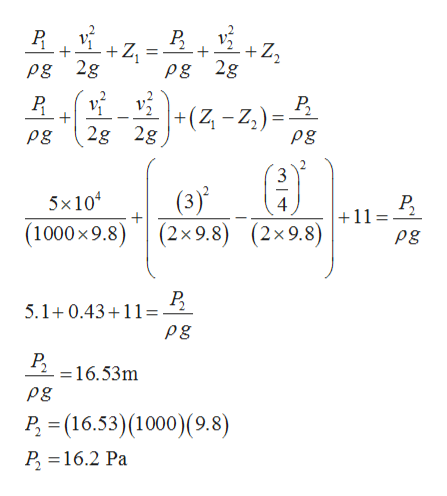# At one point in a pipeline the water's speed is 3.00 m/s and the gauge pressure is 5.00×104 Pa. Find the gauge pressure at a second point in the line, 11.0 m lower than the first, if the pipe diameter at the second point is twice that at the first.

Question

At one point in a pipeline the water's speed is 3.00 m/s and the gauge pressure is 5.00×104 Pa. Find the gauge pressure at a second point in the line, 11.0 m lower than the first, if the pipe diameter at the second point is twice that at the first.

check_circleExpert Solution
Step 1

Given,

Step 2

By applying continuity we can calculate velocity at second point,

Step 3

By applying Bernoulli&r...help_outlineImage TranscriptioncloseР. + +Z2 + Z Pg 2g Pg 2g Р. +(z-z) =_ 2g 2g Pg Pg 3 (3)' 0 x 9.8)(2x9.8) 5x10 Р. +11= 4 (2x9.8) Pg Р. 5.1+0.4311=- Pg =16.53m Pg P (16.53) (1000)(9.8) P 16.2 Pa + fullscreen

### Want to see the full answer?

See Solution

#### Want to see this answer and more?

Solutions are written by subject experts who are available 24/7. Questions are typically answered within 1 hour*

See Solution
*Response times may vary by subject and question
Tagged in

### Fluid Mechanics